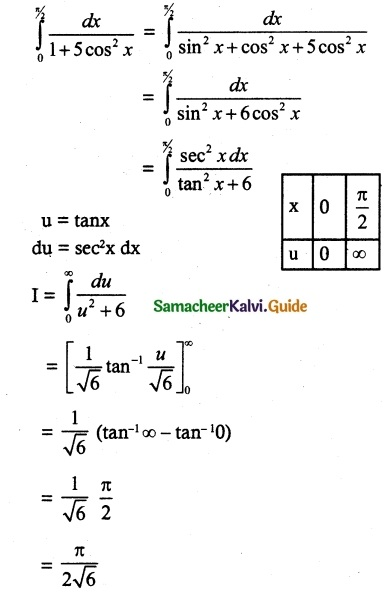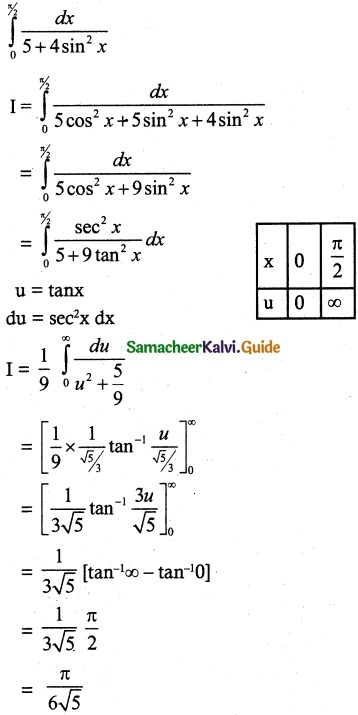Tamilnadu State Board New Syllabus Samacheer Kalvi 12th Maths Guide Pdf Chapter 9 Applications of Integration Ex 9.5 Textbook Questions and Answers, Notes.

## Tamilnadu Samacheer Kalvi 12th Maths Solutions Chapter 9 Applications of Integration Ex 9.5

Question 1.
Evaluate the following:
(i) $$\int_{0}^{π/2}$$ $$\frac{dx}{1+5cos^2x}$$
Solution:(ii) $$\int_{0}^{π/2}$$ $$\frac{dx}{5+4sin^2x}$$
Solution: